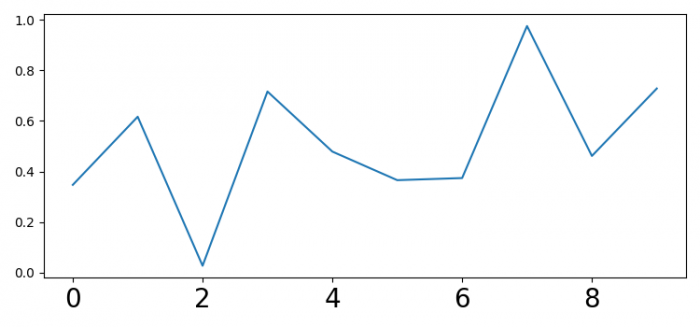# How do I change the font size of the scale in Matplotlib plots?

To change the font size of the scale in Matplotlib, we can use labelsize in the tick_params() method.

## Steps

• Set the figure size and adjust the padding between and around the subplots.
• Create a figure and a set of subplots.
• Plot x data points using plot() method.
• To change the font size of the scale in matplotlib, we can use labelsize in the ticks_params()method.
• To display the figure, use show() method.

## Example

import numpy as np
from matplotlib import pyplot as plt

plt.rcParams["figure.figsize"] = [7.50, 3.50]
plt.rcParams["figure.autolayout"] = True

fig, ax = plt.subplots()
x = np.random.rand(10)
ax.plot(x)
ax.tick_params(axis='x', labelsize=20)

plt.show()

## Output